# How to Count Blank and Non Blank Cells in Microsoft Excel

In this article, you will learn how to count the blank and how to count not blank cells in Microsoft Excel. In Excel we have functions to count the blank cells and non-blank cells.

COUNTBLANK: - When we want to count blank cells only within a range then we  use COUNTBLANK functions.

Syntax:-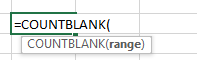COUNTA: - This function we use to count the cells within a range which is not empty.

Syntax:-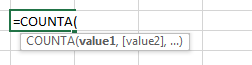Count Blank Cells

Let’s take an example:-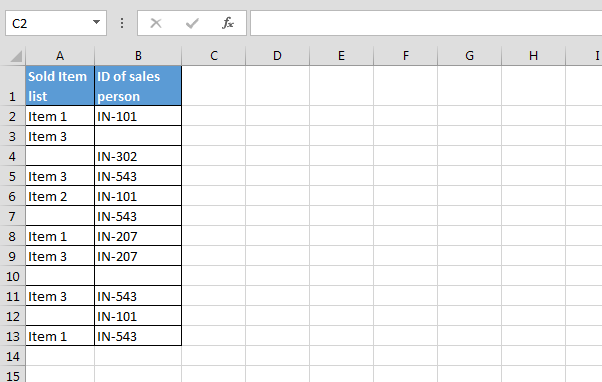First we want to calculate the blank cells in Column A, follow below given steps:-

• Enter the formula in cell C2
• =COUNTBLANK(A2:A13)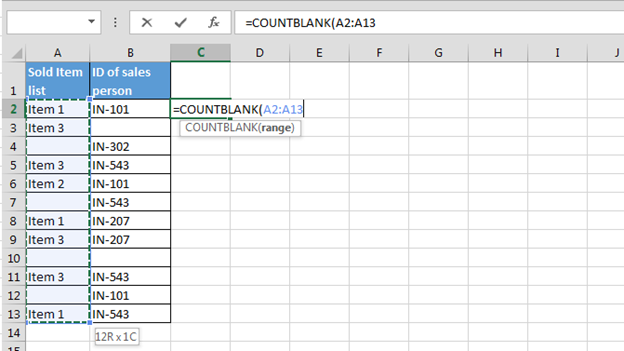• Press Enter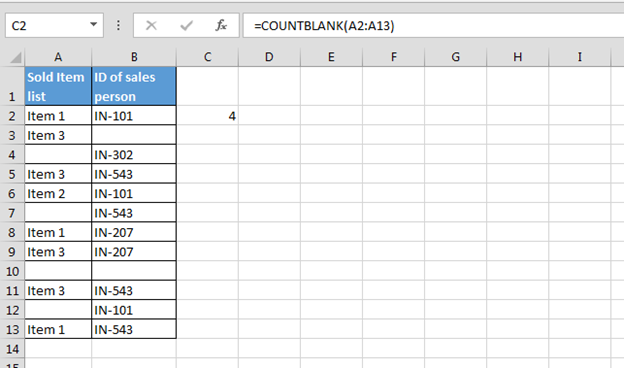Now we count the blank cells in 2 columns together, follow below given steps:-

• Enter the formula in cell C3
• =COUNTBLANK(A2:B13)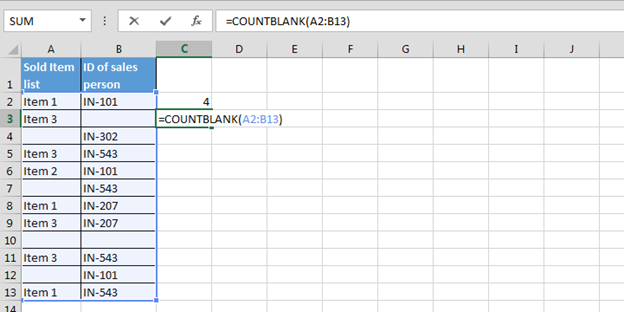• Press Enter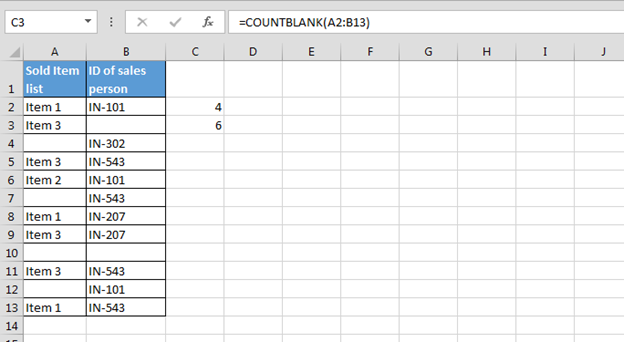Count Non-Blank Cells

Let’s take an example:-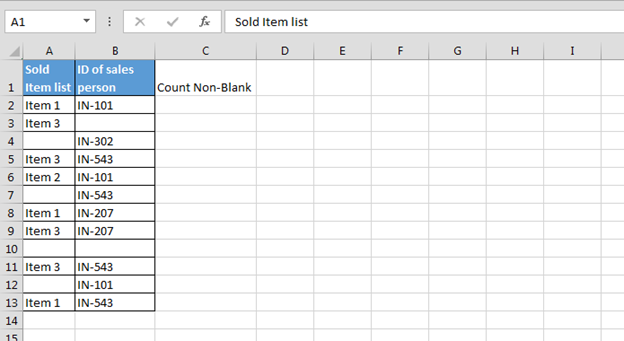First we want to calculate the blank cells in Column A, follow below given steps:-

• Enter the formula in cell C2
• =COUNTA(A2:A13)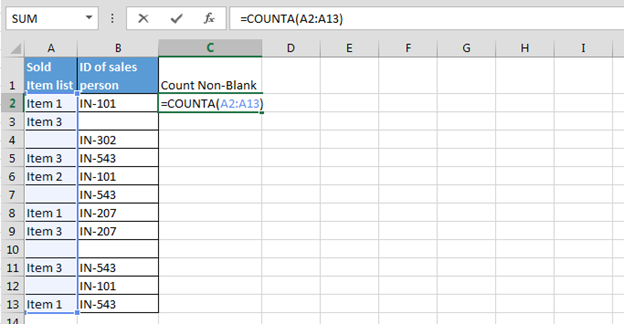• Press Enter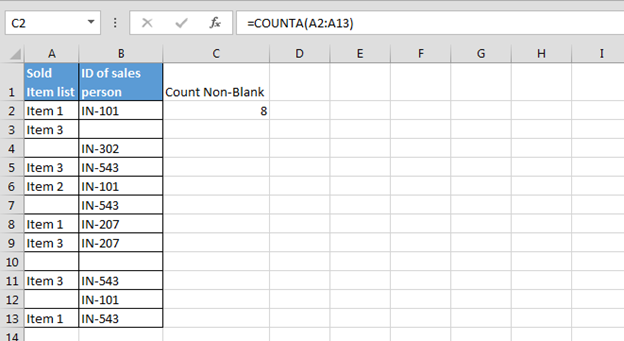Now we count the blank cells in 2 columns together, follow below given steps:-

• Enter the formula in cell C3
• =COUNTA(A2:B13)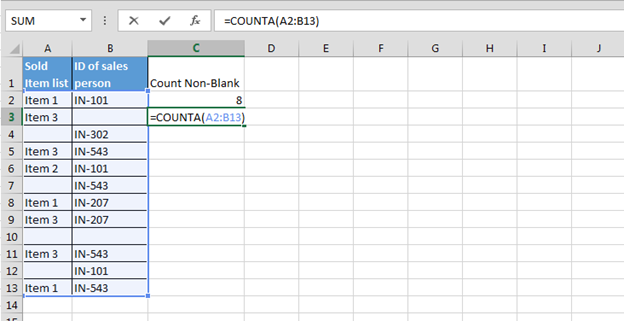• Press Enter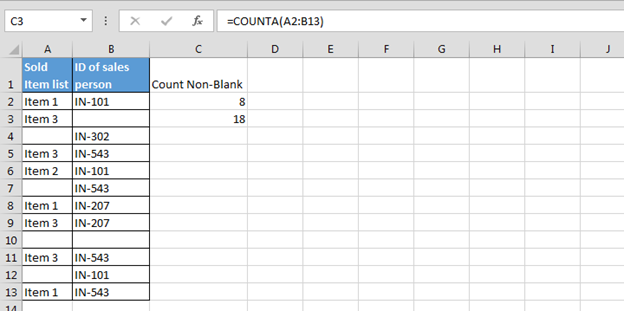Key take away:-

1.